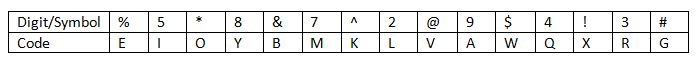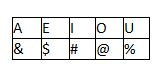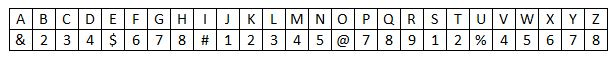Reasoning Questions – Coding And Decoding Set 28

Hello Aspirants.

Welcome to Online Reasoning Section in AffairsCloud.com. Here we are creating question sample in Coding and Decoding, which is common for all competitive exams. We have included Some questions that are repeatedly asked in bank exams!!!

(Directions 1 – 5): Given below are the codes for the digits/symbols. Study the conditions given below and answer the questions that follow.Conditions:
1. If both the first and last elements are prime number, then interchange their codes.
2. If the second element is an even digit and the fourth element is a symbol, then code both of them with the code of the fourth element.
3. If the first element is a perfect square and the last element is a symbol, then code both of them as Z.
4. If the third element is an even prime and the fifth element is an odd prime, then code both of them as S.

1. %&2#[email protected]
1) EBSVSG
2) EBSGSV
3) EDSGSV
4) GBSVSB
5) EBLGMV
Solution:
It satisfies the condition number 4.
Hence, the code is EBSGSV.

2. 3*8!#5
1) IOXYGR
2) ROYXGI
3) IOYXGI
4) IOYXGR
5) ROVXGR
Solution:
It satisfies the condition number 1.
Hence, the code is IOXYGR.

3. 9%72^@
1) ZEMLKZ
2) ZWMLKZ
3) ZEMLKV
4) AEMLKZ
5) WAMLZK
Solution:
It satisfies condition number 3.
Hence, the code is ZEMLKZ.

4. @4#&92
1) VBGABL
2) BVGBAL
3) VBGBAL
4) VQGBAL
5) None of these
Solution:
It satisfies the condition number 2.
Hence, the code is VBGBAL.

5. 8#9^3%
1) YGAKRM
2) YGARKE
3) GAYERK
4) KAYGRA
5) None of these
Solution:
It does not satisfy any of the given conditions.
Hence, the code is YGAKRE.

(Directions 6 – 10): In each of the following questions given below, a group of digits/letters is given followed by four combinations of symbols numbered A, B, C and D. You have to find out which of the following four combinations correctly represent the group of digits/letters based on the symbol codes and the conditions given below. If none of the combinations matches, choose ‘None of these’ as your answer.And the rest of the letters will be coded as the sum of the digits of the numerical position of them in the alphabetical series.

Condition 1:
If the sum of the numbers in the code is a multiple of 3 then the first two elements of the code are to be interchanged.
Condition 2:
If the product of the first and last element in the code is a multiple of 5 then the code will be written in the reversed order.
Condition 3:
If there are more than two symbols in the code then the code will be written in the reversed order except for first and the last element.

1. What would be the code of the word “ANSWER”?
1) &515#9
2) &515\$9
3) &\$5159
4) &\$9515
5) None of these
Solution:Code for the word “ANSWER” is: ‘&515\$9’Condition 3 is applied here: ‘&\$5159’.

2. What would be the code of the word “UMBRELLA”?
1) %429\$33&
2) %[email protected]
3) 4%29\$33&
4) 4%[email protected]
5) None of these
Solution:Code for the word “UMBRELLA” is: ‘%429\$33&’Condition 1 is applied here: ‘4%29\$33&’

3. What would be the code of the word “BLOCK”?
1) [email protected]
2) 23%32
3) [email protected]
4) [email protected]
5) None of these
Solution:Code for the word “BLOCK” is: ‘[email protected]
No condition is applied here.
So code for “BLOCK” is ‘[email protected]’.

4. What would be the code of the word “LAUGHTER”?
1) 3&%782#9
2) 3\$287%&9
3) 3&%782\$7
4) 3#[email protected]&9
5) 3&%782\$9
Solution:Code for the word “LAUGHTER” is: ‘3&%782\$9’Condition 3 is applied here.
So, code for “LAUGHTER” is ‘3\$287%&9’.

5. What would be the code of the word “PHOTO”?
1) 72&8&
2) [email protected]@
3) 78%2%
4) [email protected]@
5) None of these